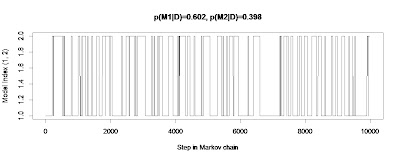## Sunday, February 26, 2012

### Autocorrelation in transdimensional MCMC with pseudopriorModel-index chain can be badly autocorrelated even with pseudopriors.
Chapter 10 of the book discusses model comparison by transdimensional MCMC in JAGS/BUGS. There can be extreme autocorrelation in the model index. The autocorrelation can be reduced by using pseudopriors. But, even with pseudopriors, the autocorrelation in the model index can still be severe. The immediate consequence is that the estimate of the model-index posterior probability can be very unstable. You may notice this instability if you run the program of Exercise 10.3 several times in JAGS, which generates a different MCMC chain every time it is run, unlike BUGS. How can this problem of unstable model-index estimates be solved?

(N.B.: Chains for continuous parameters within models can be well mixed and stable, even when the chain for the model index is badly autocorrelated.)

* Candidate Answer 1: Just use very long chains. In principle this is fine. In practice, even a moderately long chain can exceed a computer's memory. For example, I ran the model of Exercise 10.3, which has about 180 parameters, for 100,000 steps, and my aging desktop couldn't handle it. (Don't know if it was a hardware or a software memory constraint.) Therefore, this is one of those cases in which thinning is useful -- because of computer constraints, not because it reduces variability in the estimate. Run the chain a very long time, but thin it so only a fraction of the chain is stored.

* Candidate Answer 2: Instead of transdimensional MCMC, estimate p(D|M) directly within each model. One approach to doing this is provided in Section 7.3.3 (p. 137) of the book. This method requires selecting a function h(parameters) that mimics the posterior. This is much like the creation of a pseudoprior in transdimensional MCMC. The book does not discuss how to construct h(parameters) for complex models. Essentially, it is the product of the pseudopriors at the top level of the model, times the conditional probabilities for the parameter dependencies, times the likelihood: h(parameters) = p(D|param) * p(param|hyperparam) * pseudoprior(hyperparam).

* Candidate Answer 3: Use some other method to estimate p(D|M) directly within each model. See, for example, Ch. 11 of Ntzoufras (2009).

* Candidate Answer 4: Don't do model comparison, and instead just look at the parameter estimates within the more elaborate model. If you are considering nested models, the more elaborate model probably tells you everything the model comparison tells you, and more (as is discussed at length in the book, e.g., Section 10.3).